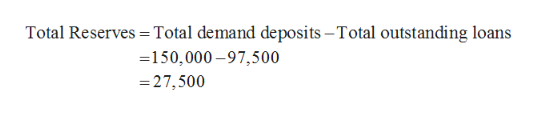# Suppose that Second Republic Bank currently has \$150,000 in demand deposits and \$97,500 in outstanding loans. The Federal Reserve has set the reserve requirement at 10%.

Question
111 views

Suppose that Second Republic Bank currently has \$150,000 in demand deposits and \$97,500 in outstanding loans. The Federal Reserve has set the reserve requirement at 10%.

check_circle

Step 1

The bank has the demand deposits of \$150,000 and the outstanding loan amount of \$97,500. It is also said that the required reserves of the bank is 10 percent. Thus, the total reserves of the bank, required reserves of the bank, and excess reserves of the bank, Money multiplier value and the size of the increase in money supply in the economy can be calculated from these details about the bank.

Step 2

The total reserves are the amount that the bank has kept without lending to the public in the form of loans to the public. Thus, it is the difference between the total demand deposits and the total outstanding loans. The total demand deposits are \$150,000 and the total outstanding loans are \$97,500. Thus, the total reserves can be calculated as follows:help_outlineImage TranscriptioncloseTotal Reserves =Total demand deposits – Total outstanding loans =150,000 – 97,500 = 27,500 fullscreen
Step 3

The required reserve of the bank is 10 percent. The required reserve is the percent of the demand deposits that the bank has to keep with it without using for loan creation in the...

### Want to see the full answer?

See Solution

#### Want to see this answer and more?

Solutions are written by subject experts who are available 24/7. Questions are typically answered within 1 hour.*

See Solution
*Response times may vary by subject and question.
Tagged in